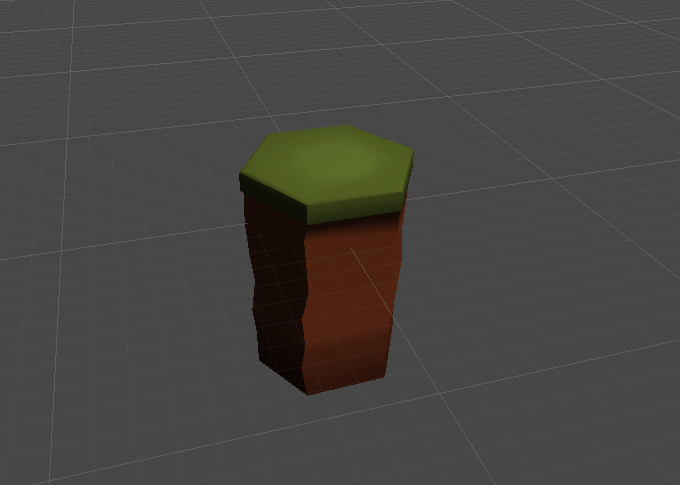# Hex Object Snapping

I’m trying to snap hexagon objects side by side with a very tiny gap so i can quickly build levels but I’m not sure how to do this.Let’s have fun with some math :

First, you need the length of the segments going from the center of a shape to the middle of the edges. Each edge form a triangle with the center as third points and with all the angles at 60°. That means 2 rectangular triangles per edge → 1 extremity, center of the edge, center of the hex. Conclusion, the segment’s length is Cos(pi / 6) / radius.

Now, to figure out the relative position of the hex to snap, I suggest Quaternaion.AngleAxis(x, Vector3.up) * Vector3.forward * segmentLength, x being 30, 90, 150, 210, 270 or 330.

It’s actually quite simple. Take a look at this picture:As you can first you need to know the radius of your hex tile. That is half of the list between two opposed corners. The two axis need different snap settings. In my example you need 3/2 the radius for clamping on the x axis and sin(60) times the radius on the y axis.

If you want a little gap, just add s bit to the radius. However i would suggest to “not” have a gap since that could confuse you even more when it comes to calculating the tile position in code.

edit
I forgot to mention that your tiles pivot should be at the center of the tileAlso that does only work for regular hexagons
Also keep in mind that not every snap position is a position in the hex grid, but all hex-grid-positions are in the snapgrid.

second edit
This solution is ment for use in the Unity editor. If you want to do that in game it’s better to calculate the real hex tile positions manually. However I understand the question that you just want a guide / helper to positioning your hex tiles in the editor, right?

third edit

Here’s another example in concrete numbers:

Just assume your tile radius is 10 so the diameter is 20

To get the grid interval for X you just multiply 10 by 3/2 which is 15

To get the grid interval for Z you multiply 10 with sin(60°)

sin(60°) is a constant which is 0.86602540378443864676372317075294 (that’s how long the windows calculator displays the number)

So for Z you would use an interval of 8.6602540378443864676372317075294

If you add a little fraction to the radius to get a gap it would look like this:

``````   new radius   = 10 + 0.1        = 10.1
X Snap       = 10.1 * 3/2      = 15.15
Z Snap       = 10.1 * sin(60)  = 8.7468565782228303323136040246047
``````